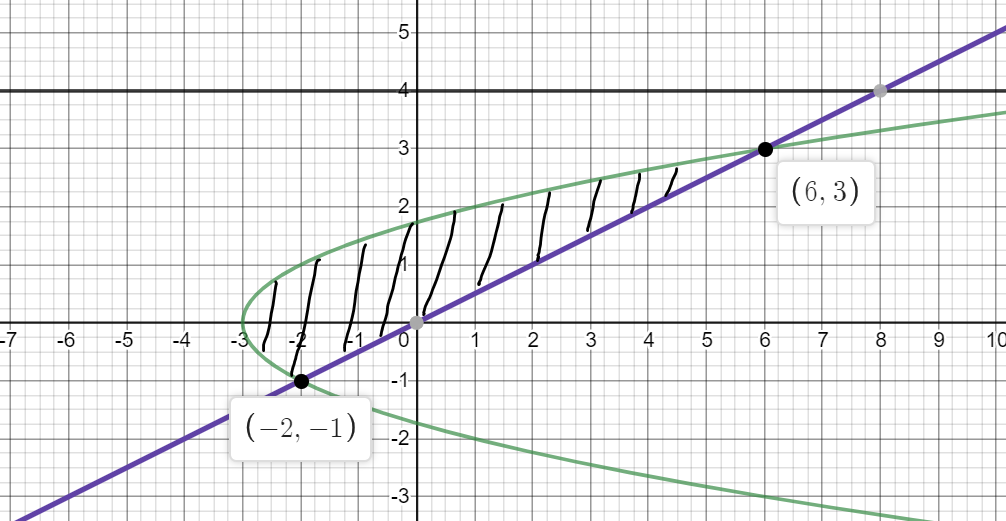# find the volume of solid obtain the by rotating the region enclosed by the by the curves about the given axis. x = y2 − 3, x = 2y, axis y = 4

Question
1 views

find the volume of solid obtain the by rotating the region enclosed by the by the curves about the given axis. x = y2 − 3, x = 2y, axis y = 4

check_circle

Step 1

We graph the region using a graphing calculator...

### Want to see the full answer?

See Solution

#### Want to see this answer and more?

Solutions are written by subject experts who are available 24/7. Questions are typically answered within 1 hour.*

See Solution
*Response times may vary by subject and question.
Tagged in